The GRE® General Test

# Overview of the Quantitative Reasoning Measure

The Quantitative Reasoning measure of the GRE General Test assesses your:

• basic mathematical skills
• understanding of elementary mathematical concepts
• ability to reason quantitatively and to model and solve problems with quantitative methods.

## Content areas covered

Some of the Quantitative Reasoning questions are posed in real-life settings, while others are posed in purely mathematical settings. Many of the questions are "word problems," which must be translated and modeled mathematically. The skills, concepts and abilities are assessed in the four content areas below.

• Arithmetic topics, including:
• properties and types of integers, such as divisibility, factorization, prime numbers, remainders and odd and even integers
• arithmetic operations, exponents and roots
• concepts such as estimation, percent, ratio, rate, absolute value, the number line, decimal representation and sequences of numbers
• Algebra topics, including:
• operations with exponents
• factoring and simplifying algebraic expressions
• relations, functions, equations and inequalities
• solving linear and quadratic equations and inequalities
• solving simultaneous equations and inequalities
• setting up equations to solve word problems
• coordinate geometry, including graphs of functions, equations and inequalities, intercepts and slopes of lines
• Geometry topics, including:
• parallel and perpendicular lines
• circles
• triangles, including isosceles, equilateral and 30°-60°-90° triangles
• other polygons
• congruent and similar figures
• 3-dimensional figures
• area
• perimeter
• volume
• the Pythagorean theorem
• angle measurement in degrees

The ability to construct proofs is not tested.

• Data analysis topics, including:
• basic descriptive statistics, such as mean, median, mode, range, standard deviation, interquartile range, quartiles and percentiles
• interpretation of data in tables and graphs, such as line graphs, bar graphs, circle graphs, boxplots, scatterplots and frequency distributions
• elementary probability, such as probabilities of compound events and independent events
• conditional probability
• random variables and probability distributions, including normal distributions
• counting methods, such as combinations, permutations and Venn diagrams

These topics are typically taught in high school algebra courses or introductory statistics courses.

Inferential statistics is not tested.

The content in these areas includes high school mathematics and statistics at a level that is generally no higher than a second course in algebra. It doesn’t include trigonometry, calculus or other higher-level mathematics. The Math Review (PDF) provides detailed information about the content of the Quantitative Reasoning measure.

## Symbols, terminology, conventions and assumptions

The mathematical symbols, terminology and conventions used in the Quantitative Reasoning measure are standard at the high school level. For example, the positive direction of a number line is to the right, distances are nonnegative and prime numbers are greater than 1. Whenever nonstandard notation is used in a question, it is explicitly introduced in the question.

In addition to conventions, there are some important assumptions about numbers and figures that are listed in the Quantitative Reasoning section directions:

• All numbers used are real numbers.
• All figures are assumed to lie in a plane unless otherwise indicated.
• Geometric figures, such as lines, circles, triangles, and quadrilaterals, are not necessarily drawn to scale. Don’t assume quantities such as lengths and angle measures are as they appear in a figure. You should assume, however, that:
• lines shown as straight are actually straight
• points on a line are in the order shown
• all geometric objects are in the relative positions shown

For questions with geometric figures, you should base your answers on geometric reasoning, not on estimating or comparing quantities by sight or by measurement.

• The following are drawn to scale. You can read, estimate or compare quantities and data values by sight or by measurement:
• coordinate systems, such as xy-planes and number lines
• graphical data presentations such as bar graphs, circle graphs and line graphs

## Question types and Data Interpretation sets

The Quantitative Reasoning measure has four types of questions:

• Quantitative Comparison Questions
• Multiple-choice Questions — Select One Answer Choice
• Multiple-choice Questions — Select One or More Answer Choices
• Numeric Entry Questions

Each question appears either independently as a discrete question or as part of a set of questions called a Data Interpretation set. All questions in a Data Interpretation set are based on the same data presented in tables, graphs or other displays of data.

These questions ask you to compare two quantities — Quantity A and Quantity B — and then determine which of the following statements describes the comparison.

• Quantity A is greater.
• Quantity B is greater.
• The two quantities are equal.
• The relationship cannot be determined from the information given.

1. Become familiar with the answer choices. Quantitative Comparison questions always have the same answer choices, so get to know them, especially the last choice, "The relationship cannot be determined from the information given." Never select this last choice if it’s clear the values of the two quantities can be determined by computation. Also, if you determine that one quantity is greater than the other, make sure you carefully select the corresponding choice and don’t reverse the first two choices.
2. Avoid unnecessary computations. Don't waste time performing needless computations to compare the two quantities. Simplify, transform or estimate one or both of the given quantities only as needed to compare them.
3. Remember that geometric figures aren’t necessarily drawn to scale. If any aspect of a given geometric figure is not fully determined, try to redraw the figure, keeping those aspects that are completely determined by the given information fixed but changing the aspects of the figure that are not determined. Examine the results. What variations are possible in the relative lengths of line segments or measures of angles?
4. Plug in numbers. If one or both quantities are algebraic expressions, you can substitute easy numbers for the variables and compare the resulting quantities in your analysis. Consider all kinds of appropriate numbers before you give an answer: e.g., zero, positive and negative numbers, small and large numbers, fractions and decimals. If you see that Quantity A is greater than Quantity B in one case and Quantity B is greater than Quantity A in another case, choose "The relationship cannot be determined from the information given."
5. Simplify the comparison. If both quantities are algebraic or arithmetic expressions and you can’t easily see a relationship between them, try to simplify the comparison. Try a step-by-step simplification that is similar to the steps involved when you solve the equation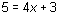for x,  or similar to the steps involved when you determine that the inequality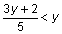is equivalent to the simpler inequality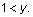Begin by setting up a comparison involving the two quantities: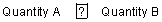where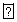is a placeholder that could represent the relationship greater than (>), less than (<), or equal to (=), or could represent the fact that the relationship can’t be determined from the information given. Then try to simplify the comparison, step by step, until you can determine a relationship between simplified quantities. For example, you may conclude after the last step thatrepresents equal to (=). Based on this conclusion, you may be able to compare Quantities A and B. To understand this strategy more fully, see sample questions 6 to 9.

These multiple-choice questions ask you to select only one answer choice from a list of five choices.

• Reread the question carefully — you may have missed an important detail or misinterpreted some information.
• Check your computations — you may have made a mistake, such as mis-keying a number on the calculator.
• Reevaluate your solution method — you may have a flaw in your reasoning.
2. Examine the answer choices. In some questions you’re asked explicitly which of the choices has a certain property. You may have to consider each choice separately or you may be able to see a relationship between the choices that will help you find the answer more quickly. In other questions, it may be helpful to work backward from the choices, for example, by substituting the choices in an equation or inequality to see which one works. However, be careful, as that method may take more time than using reasoning.
3. For questions that require approximations, scan the answer choices to see how close an approximation is needed. (This may be helpful for other questions too, as it can help you get a better sense of what the question is asking.) For some questions, you may have to carry out all computations exactly and round only your final answer in order to get the required degree of accuracy. In others, estimation is sufficient and will help you avoid spending time on long computations.

These multiple-choice questions ask you to select one or more answer choices from a list of choices. The question may or may not specify the number of choices to select.

1. Note whether you’re asked to indicate a specific number of answer choices or all choices that apply. In the latter case, be sure to consider all of the choices, determine which ones are correct, and select all of those and only those choices. Note that there may be only one correct choice.
2. In some questions that involve conditions that limit the possible values of the numerical answer choices, it may be efficient to determine the least and/or the greatest possible value. Knowing the least and/or greatest possible value may enable you to quickly determine all the correct choices.
3. Avoid lengthy calculations by recognizing and continuing numerical patterns.

These questions ask you either to enter your answer as an integer or a decimal in a single answer box or as a fraction in two separate boxes — one for the numerator and one for the denominator. You’ll use the computer mouse and keyboard to enter your answer.

1. Make sure you answer the question that’s asked. Since there are no answer choices to guide you, read the question carefully and make sure you provide the type of answer required. Sometimes there will be labels before or after the answer box to indicate the appropriate type of answer. Pay special attention to units such as feet or miles, to orders of magnitude such as millions or billions, and to percents as compared with decimals.
2. If you’re asked to round your answer, make sure you round to the required degree of accuracy. For example, if an answer of 46.7 is to be rounded to the nearest integer, you need to enter the number 47. If your solution strategy involves intermediate computations, carry out all computations exactly and round only your final answer in order to get the required degree of accuracy. If no rounding instructions are given, enter the exact answer.
3. Examine your answer to see if it’s reasonable with respect to the information given. You may want to use estimation or another solution path to double-check your answer.

Data Interpretation questions are grouped together and refer to the same table, graph or other data presentation. These questions ask you to interpret or analyze the given data. The types of questions may be Multiple-choice (both types) or Numeric Entry.

1. Scan the data presentation briefly to see what it’s about, but don’t spend time studying all of the information in detail. Focus on those data aspects that are necessary to answer the questions. Pay attention to:
• the axes and scales of graphs
• the units of measurement or orders of magnitude (such as billions) that are given in the titles, labels and legends
• any notes that clarify the data
2. When graphical data presentations such as bar graphs and line graphs are shown with scales, you should read, estimate, or compare quantities by sight or by measurement, according to the corresponding scales. For example, use the relative sizes of bars or sectors to compare the quantities they represent, but be aware of broken scales and of bars that don’t start at 0.
3. Answer questions only on the basis of the data presented, everyday facts (such as the number of days in a year) and your knowledge of mathematics. Don’t make use of specialized information you may recall from other sources about the particular context on which the questions are based unless the information can be derived from the data presented.

## Problem-solving steps

In addition to the tips for answering in the question type sections above, there are also some general problem-solving steps and strategies you can employ. Questions in the Quantitative Reasoning measure ask you to model and solve problems using quantitative, or mathematical, methods. Generally, there are three basic steps in solving a mathematics problem:

Read the statement of the problem carefully to make sure you understand the information given and the problem you are being asked to solve.

• Some information may describe certain quantities.
• Quantitative information may be given in words or mathematical expressions, or a combination of both.
• You may need to read and understand quantitative information in data presentations, geometric figures or coordinate systems.
• Other information may take the form of formulas, definitions or conditions that must be satisfied by the quantities. For example, the conditions may be equations or inequalities, or may be words that can be translated into equations or inequalities.

In addition to understanding the information you are given, make sure you understand what you need to accomplish in order to solve the problem. For example, what unknown quantities must be found? In what form must they be expressed?

Solving a mathematics problem requires more than understanding a description of the problem (the quantities, the data, the conditions, the unknowns and all other mathematical facts related to the problem). It also requires determining what mathematical facts to use and when and how to use those facts to develop a solution to the problem. It requires a strategy.

Mathematics problems are solved by using a wide variety of strategies, and there may be different ways to solve a given problem. Develop a repertoire of problem-solving strategies and a sense of which strategies are likely to work best in solving particular problems. Attempting to solve a problem without a strategy may lead to a lot of work without producing a correct solution.

After you determine a strategy, carry it out. If you get stuck, check your work to see if you made an error in your solution. Maintain a flexible, open mindset. If you check your solution and can’t find an error, or if your solution strategy is simply not working, look for a different strategy.

When you arrive at an answer, check that it’s reasonable and computationally correct.

• Is your answer reasonable in the context of the question? Checking that an answer is reasonable can be as simple as recalling a basic mathematical fact and checking whether your answer is consistent with that fact. For example, the probability of an event must be between 0 and 1, inclusive, and the area of a geometric figure must be positive. You may be able to use estimation to check that your answer is reasonable. For example, if your solution involves adding three numbers, each of which is between 100 and 200, estimating the sum tells you that the sum must be between 300 and 600.
• Did you make a computational mistake in arriving at your answer or a key-entry error using the calculator? Check for errors in each step in your solution. Or you may be able to check directly that your solution is correct. For example, if you solve an equation for x, substitute your answer into the equation to make sure it’s correct.

## Strategies

There are no set rules — applicable to all mathematics problems — to determine the best strategy. The ability to determine a strategy that will work grows as you solve more and more problems. Download the Sample Questions for a list of 14 useful strategies you can employ, along with one or two sample questions that illustrate how to use each strategy.

## Calculator use

You’re provided with a basic on-screen calculator on the Quantitative Reasoning measure. Sometimes the computations you need to do to answer a question in the Quantitative Reasoning measure are somewhat time-consuming, like long division, or they involve square roots. Although the calculator can shorten the time it takes to perform computations, keep in mind that the calculator provides results that supplement, but don’t replace, your knowledge of mathematics. You’ll need to use your mathematical knowledge to determine whether the calculator's results are reasonable and how the results can be used to answer a question.

Here are some general guidelines for calculator use in the Quantitative Reasoning measure:

• Most of the questions don't require difficult computations, so don't use the calculator just because it's available.
• Use it for calculations that you know are tedious, such as long division, square roots, and addition, subtraction, or multiplication of numbers that have several digits.
• Avoid using it for simple computations that are quicker to do mentally, such as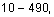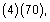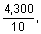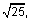and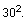• Avoid using it to introduce decimals if you’re asked to give an answer as a fraction.
• You may be able to answer some questions more quickly by reasoning and estimating than by using the calculator.
• If you use the calculator, estimate the answer beforehand so you can determine whether the calculator's answer is "in the ballpark." This may help you avoid key-entry errors.